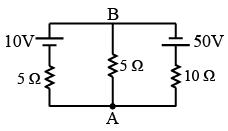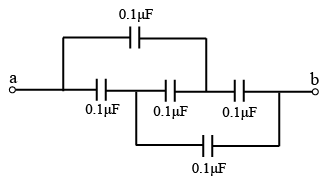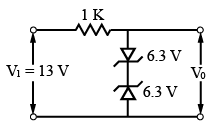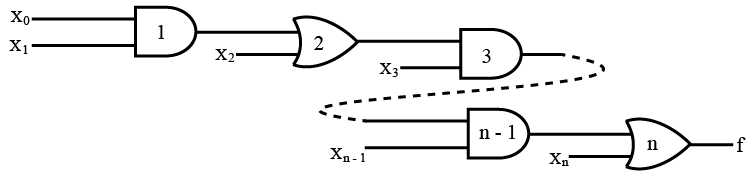# ISRO Scientist or Engineer Electronics 2006

Instructions

For the following questions answer them individually

Question 11

# For the circuit shown in the given figure, the voltage $$V_{AB}$$ isQuestion 12

# The equivalent Capacitance across 'ab' will beQuestion 13

# The transfer function, $$T(s) = \frac{s}{s + a}$$ is that of aQuestion 14

# A particular current is made up of two components: a 10 A dc and a sinusoidal current of peak value of 1.414 A. The average value of the resultant current isQuestion 15

# By doubling the sampling frequencyQuestion 16

# The output voltage ($$v_o$$) of the circuit shown in the given figure isQuestion 17

# Assuming that only the X and Y logic inputs are available and their complements $$\overline{X}$$ and $$\overline{Y}$$ are not available, what is the minimum number of two-input NAND gates requires to implement $$X \oplus Y$$Question 18

# In the given network of AND and OR gates f can be written as:Question 19

# A Pulse train with a frequency of 1MHz is counted using a modulo 1024 ripple-counter built with J-K flip-flops. For proper operation of the counter the maximum permissible propagation delay per flip-flop stage isQuestion 20

# The A/D converter used in a digital voltmeter could be (1) successive approximation type (2) Flash converter type (3) Dual slope converter type. The correct sequence in the increasing order of their conversion times isOR